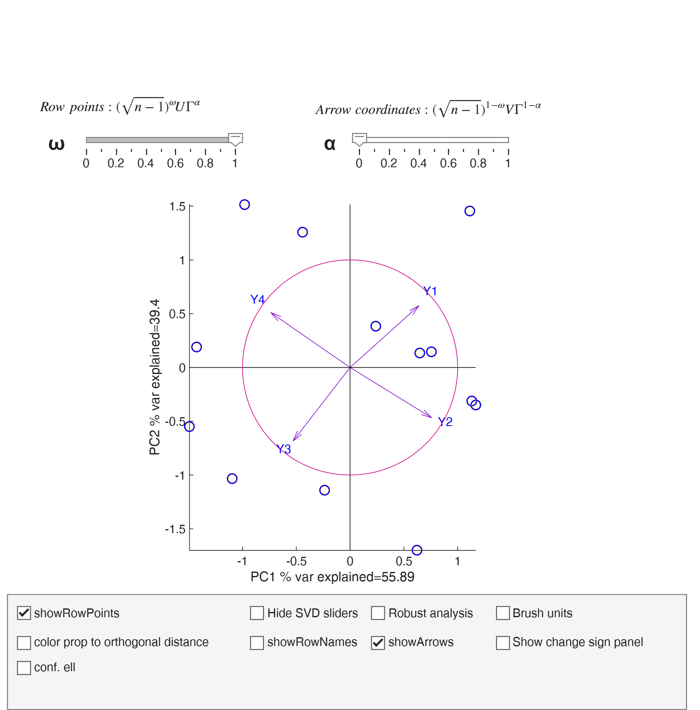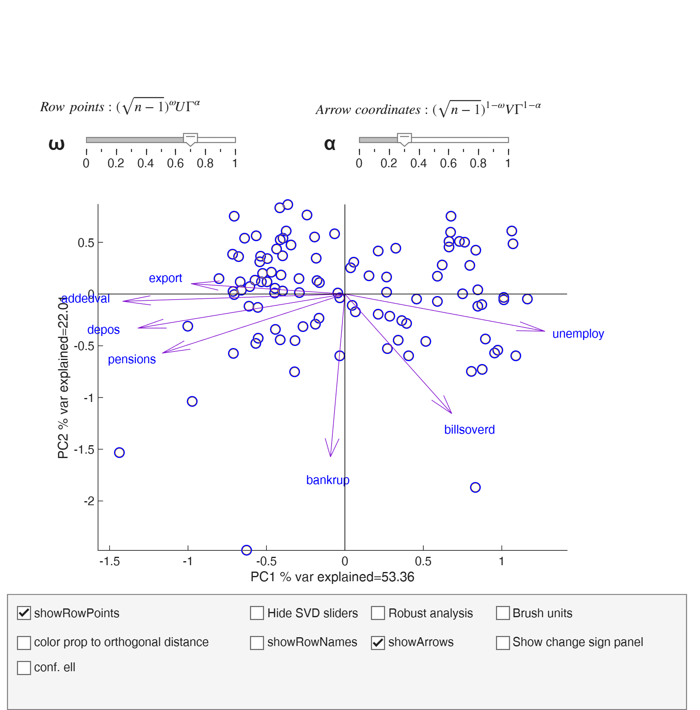biplotFS

biplotFS calls biplotAPP.mlapp to show a dynamic biplot

Description

The svd of $Z=zscore(Y)$ $Z= U \Gamma^* V^T$ and its best rank two approximation $Z \approx U_{(2)} \Gamma_{(2)}^* V_{(2)}^T$

where $U_{(2)}$ is $n \times 2$ matrix (first two columns of $U$) and $V_{(2)}$ is $p \times 2$ (first two columns of matrix $V$, $\Gamma_{(2)}^*$ is a $2 \times 2$ diagonal matrix which contains the frist two largest singular values of matrix $Z$ (square root of the eigenvalues of matrix $Z^TZ=(n-1)R$) where $R$ is the correlation matrix.

We can also write

$\frac{Z}{\sqrt{n-1}} \approx U_{(2)}\frac{\Gamma_{(2)}^*}{\sqrt{n-1}} V_{(2)}^T$ $Z \approx \sqrt{n-1} U_{(2)}\frac{\Gamma_{(2)}^*}{\sqrt{n-1}} V_{(2)}^T$ $Z \approx \sqrt{n-1} U_{(2)} \Gamma_{(2)} V_{(2)}^T$

where $\Gamma_{(2)} = \Gamma_{(2)}^*/ \sqrt{n-1}$.

In this way $\Gamma_{(2)}$ contains the singular values (square root of the eigenvalues) of matrix $Z^TZ/(n-1)=R$ (correlation matrix).

The last equation can be written as function of two parameters $\alpha \in [0 1]$ and $\omega \in [0 1]$

$Z \approx \left[ (\sqrt{n-1})^\omega U_{(2)} \Gamma_{(2)}^\alpha \right] [ \Gamma_{(2)}^{1-\alpha} V_{(2)}^T (\sqrt{n-1})^{1-\omega} ]$

In the dynamic biplot the $n$ row points are represented by $n \times 2$ matrix:

$\left[ (\sqrt{n-1})^\omega U_{(2)} \Gamma_{(2)}^\alpha \right]$ and the $p$ column points (arrows) through $2 \times p$ matrix $[ \Gamma_{(2)}^{1-\alpha} V_{(2)}^T (\sqrt{n-1})^{1-\omega} ]$ Note that if $\omega=1$ and $\alpha=0$ row points are $\sqrt{n-1} U_{(2)}= Z V_{(2)} \Gamma_{(2)}^{-1}$

that is row points are the standardized principal components scores.

$cov (\sqrt{n-1} U_{(2)}) = I_2= \left( \begin{array}{cc} 1 & 0 \\ 0 & 1 \\ \end{array} \right)$ Column points are $\Gamma_{(2)} V_{(2)}^T$

that is the arrows are associated with the correlations between the variables and the first two principal components The length of the arrow is exactly equal to the communality of the asoociated variable.

In this case the unit circle is also shown on the screen and option axis equal is set.

On the other hand, if $\omega=1$ and $\alpha=1$ the $n$ rows of $Z$ (of dimension $n \times p$) can be represented using $n \times 2$ matrix:

$\sqrt{n-1} U_{(2)} \Gamma_{(2)} = Z V_{(2)}$ In this case the row points are the (non normalized) scores, that is $cov(\sqrt{n-1} U_{(2)} \Gamma_{(2)}) =cov( Z V_{(2)})= \left( \begin{array}{cc} \lambda_1 & 0 \\ 0 & \lambda_2 \\ \end{array} \right)$ With this parametrization the column points (arrows) are nothing but the coordinates of the first two eigenvectors $V_{(2)}^T$

Also in this case the unit circle is given and option axis equal is set.

In general if $\omega$ decreases, the length of the arrows increases and the coordinates of row points are squeezed towards the origin

biplotFS(Y) use of biplotFS on the ingredients dataset.

biplotFS(Y, Name, Value) use of biplotFS on the dataset referred to Italian cities.

Examples

expand alluse of biplotFS on the ingredients dataset.

% Operate on the correlation matrix (default).
% use standardized principal components (for row points) and
% correlation between variables and principal components (for column
% points, arrows)
close all
biplotFS(ingredients,'omega',1,'alpha',0);
When \omega=1 and \alpha=0
Row points represent standardized principal components sqrt(n-1)*U=ZV*\Gamma^-1
Arrows represent correlations between variables and principal components \Gamma*V
The length of the arrows is the communality of the variable
In this case the unit circle is also shownuse of biplotFS on the dataset referred to Italian cities.

biplotFS(citiesItaly)Input Arguments

Y — Input data. 2D array or table.

n x v data matrix; n observations and v variables. Rows of Y represent observations, and columns represent variables.

Missing values (NaN's) and infinite values (Inf's) are allowed, since observations (rows) with missing or infinite values will automatically be excluded from the computations.

Data Types: single|double

Name-Value Pair Arguments

Specify optional comma-separated pairs of Name,Value arguments. Name is the argument name and Value is the corresponding value. Name must appear inside single quotes (' '). You can specify several name and value pair arguments in any order as Name1,Value1,...,NameN,ValueN.

Example: 'standardize',false , 'alpha',0.6 , 'omega',1 , 'standardize',false , 'showRowNames',false , 'showArrows',false

standardize —standardize data.boolean.

Boolean which specifies whether to standardize the variables, that is we operate on the correlation matrix (default) or simply remove column means (in this last case we operate on the covariance matrix).

Example: 'standardize',false

Data Types: boolean

alpha —svd parameter.scalar.

Scalar in the interval [0 1] (see section additional details for more help). This parameter can be controllad by the corresponding sliding bar when the app is shown.

Example: 'alpha',0.6

Data Types: double

omega —svd parameter.scalar.

Scalar in the interval [0 1] (see section additional details for more help). This parameter can be controllad by the corresponding sliding bar when the app is shown.

Example: 'omega',1

Data Types: double

showRowPoints —hide or show row point.boolean.

If showRowPoints is true row points are shown in the biplot (default) else there are hidden.

Example: 'standardize',false

Data Types: boolean

showRowNames —hide or show labels of row points.boolean.

If showRowNames is true labels of row names are shown in the biplot else (default) there are hidden.

Example: 'showRowNames',false

Data Types: boolean

showArrows —hide or show arrows.boolean.

If showArrows is true arrows (associated labels) labels are shown in the biplot (default) else there are hidden.

Example: 'showArrows',false

Data Types: boolean